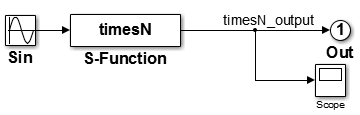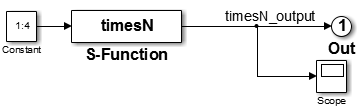## Explore Variable Names and Loop Rolling

### `timesN` Looping Tutorial Overview

Objective: This example shows how you can influence looping behavior of generated code.

Folder: `matlabroot/toolbox/rtw/rtwdemos/tlctutorial/timesN` (open)

Work with the model `sfun_xN` in `tlctutorial/timesN`. It has one source (a Sine Wave generator block), a times N gain block, an Out block, and a Scope block.

The tutorial guides you through following steps:

1. Getting Started — Set up the exercise and run the model

2. Modify the Model — Change the input width and see the results

3. Change the Loop Rolling Threshold — Change the threshold and see the results

4. More About TLC Loop Rolling — Parameterize loop behavior

### Getting Started

1. Make `tlctutorial/timesN` your current folder, so that you can use the files provided.

Note

You must use or create a working folder outside of `matlabroot` for Simulink® models you make. You cannot build models in source folders.

2. In the MATLAB® Command Window, create a MEX-file for the S-function:

`mex timesN.c`

This avoids picking up the version shipped with Simulink.

Note

An error might occur if you have not previously run ```mex -setup```.

3. Open the model file `sfun_xN`.4. View the previously generated code in `sfun_xN_grt_rtw/sfun_xN.c`. Note that no loops exist in the code. This is because the input and output signals are scalar.

### Modify the Model

1. Replace the Sine Wave block with a Constant block.

2. Set the parameter for the Constant block to 1:4, and change the top label, `model: sfun_xN`, to `model: sfun_vec`.

3. Save the edited model as `sfun_vec` (in `tlctutorial/timesN`). The model now looks like this.4. Because the Constant block generates a vector of values, this is a vectorized model. Generate code for the model and view the ```/*Model output function */``` section of `sfun_vec.c` in your editor to observe how variables and `for` loops are handled. This function appears as follows:

```/* Model output function */ static void sfun_vec_output(int_T tid) { /* S-Function Block: <Root>/S-Function */ /* Multiply input by 3.0 */ sfun_vec_B.timesN_output = sfun_vec_P.Constant_Value * 3.0; sfun_vec_B.timesN_output = sfun_vec_P.Constant_Value * 3.0; sfun_vec_B.timesN_output = sfun_vec_P.Constant_Value * 3.0; sfun_vec_B.timesN_output = sfun_vec_P.Constant_Value * 3.0; /* Outport: '<Root>/Out' */ sfun_vec_Y.Out = sfun_vec_B.timesN_output; sfun_vec_Y.Out = sfun_vec_B.timesN_output; sfun_vec_Y.Out = sfun_vec_B.timesN_output; sfun_vec_Y.Out = sfun_vec_B.timesN_output; UNUSED_PARAMETER(tid); }```

Notice that there are four instances of the code that generates model outputs, corresponding to four iterations.

5. Set the parameter for the Constant block to 1:10, and save the model.

6. Generate code for the model and view the ```/*Model output function */``` section of `sfun_vec.c` in your editor to observe how variables and `for` loops are handled. This function appears as follows:

```/* Model output function */ static void sfun_vec_output(int_T tid) { /* S-Function Block: <Root>/S-Function */ /* Multiply input by 3.0 */ { int_T i1; const real_T *u0 = &sfun_vec_P.Constant_Value; real_T *y0 = sfun_vec_B.timesN_output; for (i1=0; i1 < 10; i1++) { y0[i1] = u0[i1] * 3.0; } } { int32_T i; for (i = 0; i < 10; i++) { /* Outport: '<Root>/Out' */ sfun_vec_Y.Out[i] = sfun_vec_B.timesN_output[i]; } } UNUSED_PARAMETER(tid); }```

Notice that:

• The code that generates model outputs gets “rolled” into a loop. This occurs by default when the number of iterations exceeds 5.

• Loop index `i1` runs from 0 to 9.

• Pointer `*y0` is used and initialized to the output signal array.

### Change the Loop Rolling Threshold

The code generator creates iterations or loops depending on the current value of the Loop unrolling threshold parameter.

The default value of Loop unrolling threshold is `5`. To change looping behavior for blocks in a model:

1. On the Optimization pane of the Configuration Parameters dialog box, set Loop unrolling threshold to `12` and click `Apply`.

The parameter `RollThreshold` is now `12`. Loops will be generated only when the width of signals passing through a block exceeds 12.

Note

You cannot modify `RollThreshold` for specific blocks from the Configuration Parameters dialog box.

2. Press Ctrl+B to regenerate the output.

3. Inspect `sfun_vec.c`. It will look like this:

```/* Model output function */ static void sfun_vec_output(int_T tid) { /* S-Function Block: <Root>/S-Function */ /* Multiply input by 3.0 */ sfun_vec_B.timesN_output = sfun_vec_P.Constant_Value * 3.0; sfun_vec_B.timesN_output = sfun_vec_P.Constant_Value * 3.0; sfun_vec_B.timesN_output = sfun_vec_P.Constant_Value * 3.0; sfun_vec_B.timesN_output = sfun_vec_P.Constant_Value * 3.0; sfun_vec_B.timesN_output = sfun_vec_P.Constant_Value * 3.0; sfun_vec_B.timesN_output = sfun_vec_P.Constant_Value * 3.0; sfun_vec_B.timesN_output = sfun_vec_P.Constant_Value * 3.0; sfun_vec_B.timesN_output = sfun_vec_P.Constant_Value * 3.0; sfun_vec_B.timesN_output = sfun_vec_P.Constant_Value * 3.0; sfun_vec_B.timesN_output = sfun_vec_P.Constant_Value * 3.0; /* Outport: '<Root>/Out' */ sfun_vec_Y.Out = sfun_vec_B.timesN_output; sfun_vec_Y.Out = sfun_vec_B.timesN_output; sfun_vec_Y.Out = sfun_vec_B.timesN_output; sfun_vec_Y.Out = sfun_vec_B.timesN_output; sfun_vec_Y.Out = sfun_vec_B.timesN_output; sfun_vec_Y.Out = sfun_vec_B.timesN_output; sfun_vec_Y.Out = sfun_vec_B.timesN_output; sfun_vec_Y.Out = sfun_vec_B.timesN_output; sfun_vec_Y.Out = sfun_vec_B.timesN_output; sfun_vec_Y.Out = sfun_vec_B.timesN_output; UNUSED_PARAMETER(tid); }```
4. To activate loop rolling again, change the Loop unrolling threshold to 10 (or less) on the Optimization pane.

Loop rolling is an important TLC capability for optimizing generated code. Take some time to study and explore its implications before generating code for production requirements.

### More About TLC Loop Rolling

The following TLC `%roll` code is the `Outputs` function of `timesN.tlc`:

```%function Outputs(block, system) Output /* %<Type> Block: %<Name> */ %% /* Multiply input by %<gain> */ %assign rollVars = ["U", "Y"] %roll idx = RollRegions, lcv = RollThreshold, block, "Roller", rollVars %<LibBlockOutputSignal(0, "", lcv, idx)> = \ %<LibBlockInputSignal(0, "", lcv, idx)> * %<gain>; %endroll %endfunction %% Outputs```

#### Arguments for `%roll`

The lines between `%roll` and %`endroll` may be either repeated or looped. The key to understanding the `%roll` directive is in its arguments:

`%roll sigIdx = RollRegions, lcv = RollThreshold, block, "Roller", rollVars`
ArgumentDescription
`sigIdx`

Specify the index into a (signal) vector that is used in the generated code. If the signal is scalar, when analyzing that block of the `model.rtw` file, TLC determines that only a single line of code is required. In this case, it sets `sigIdx` to `0` so as to access only the first element of a vector, and no loop is constructed.

`lcv`

A control variable generally specified in the `%roll` directive as `lcv = RollThreshold`. `RollThreshold` is a global (model-wide) threshold with the default value of 5. Therefore, whenever a block contains more than five contiguous and rollable variables, TLC collapses the lines nested between `%roll` and `%endroll` into a loop. If fewer than five contiguous rollable variables exist, `%roll` does not create a loop and instead produces individual lines of code.

`block`

This tells TLC that it is operating on block objects. TLC code for S-functions use this argument.

`"Roller"`

This, specified in `rtw/c/tlc/roller.tlc`, formats the loop. Normally you pass this as is, but other loop control constructs are possible for advanced uses (see `LibBlockInputSignal` in Input Signal Functions).

`rollVars`

Tells TLC what types of items should be rolled: input signals, output signals, and/or parameters. You do not have to use all of them. In a previous line, `rollVars` is defined using `%assign`.

`%assign rollVars = ["U", "Y"]`
This list tells TLC that it is rolling through input signals (`U`) and output signals (`Y`). In cases where blocks specify an array of parameters instead of a scalar parameter, `rollvars` is specified as
`%assign rollVars = ["U", "Y", "P"]`

#### Input Signals, Output Signals, and Parameters

Look at the lines that appear between `%roll` and `%endroll`:

```%<LibBlockOutputSignal(0, "", lcv, idx)> = \ %<LibBlockInputSignal (0, "", lcv, idx)> * 2.0;```

The TLC library functions `LibBlockInputSignal` and `LibBlockOutputSignal` expand to produce scalar or vector identifiers that are named and indexed. `LibBlockInputSignal`, `LibBlockOutputSignal`, and a number of related TLC functions are passed four canonical arguments:

ArgumentDescription

first argument — `0`

Corresponds to the input port index for a given block. The first input port has index 0. The second input port has index 1, and so on.

second argument — `" "`

An index variable reserved for advanced use. For now, specify the second argument as an empty string. In advanced applications, you may define your own variable name to be used as an index with `%roll`. In such a case, TLC declares this variable as an integer in a location in the generated code.

third argument — `lcv`

As described previously, `lcv = RollThreshold` is set in `%roll` to indicate that a loop be constructed whenever `RollThreshold` (default value of 5) is exceeded.

fourth argument — `sigIdx`

Enables TLC to handle special cases. In the event that the `RollThreshold` is not exceeded (for example, if the block is only connected to a scalar input signal) TLC does not roll it into a loop. Instead, TLC provides an integer value for the index variable in a corresponding line of “inline” code. Whenever the `RollThreshold` is exceeded, TLC creates a `for` loop and uses an index variable to access inputs, outputs and parameters within the loop.